# OECD Crop production data analysis 1 - Import CSV file into R using read_csv() function. MAIZE has the largest cropfields and volume.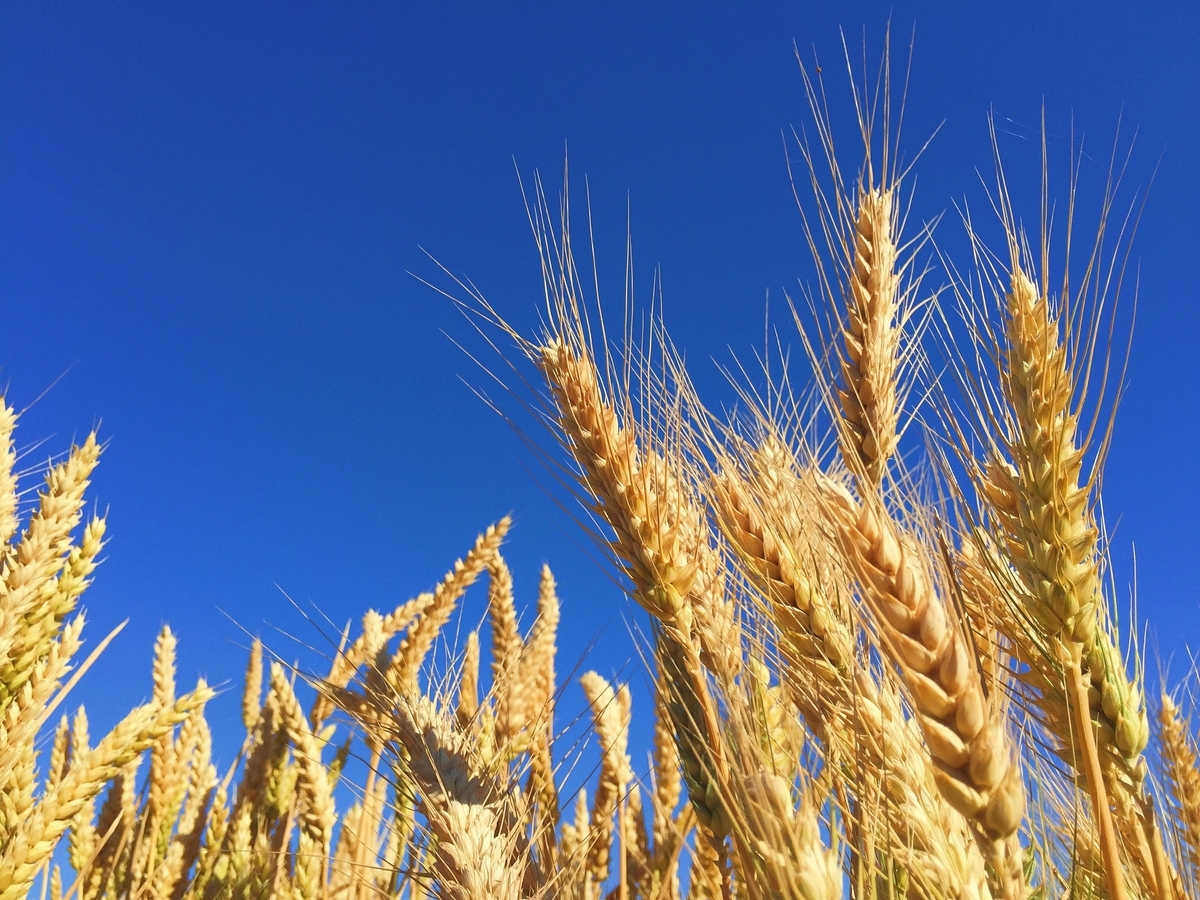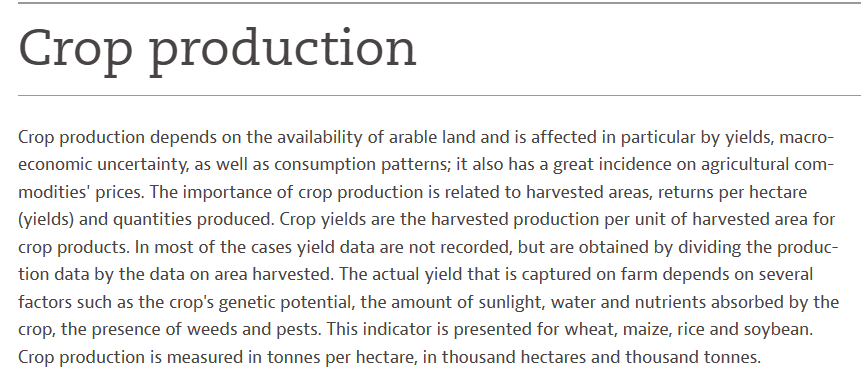In this post, I will analyzie OECD Crop production data.

Firstly, I downloaded data from "OECD (2023), Crop production (indicator). doi: 10.1787/49a4e677-en (Accessed on 01 July 2023)"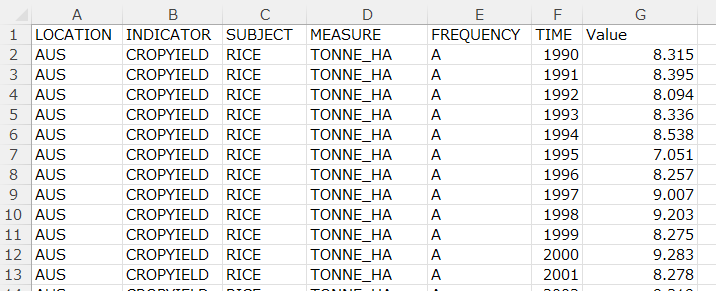I use R to analyze the data.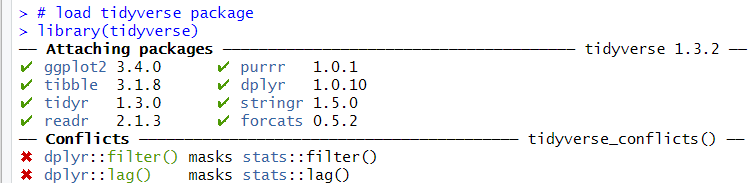Next, I use read_csv() fundtion to load the CSV file data into R.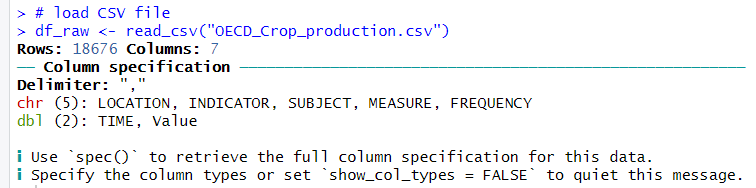Then, I use skim() function from skimr package to check each varibales data.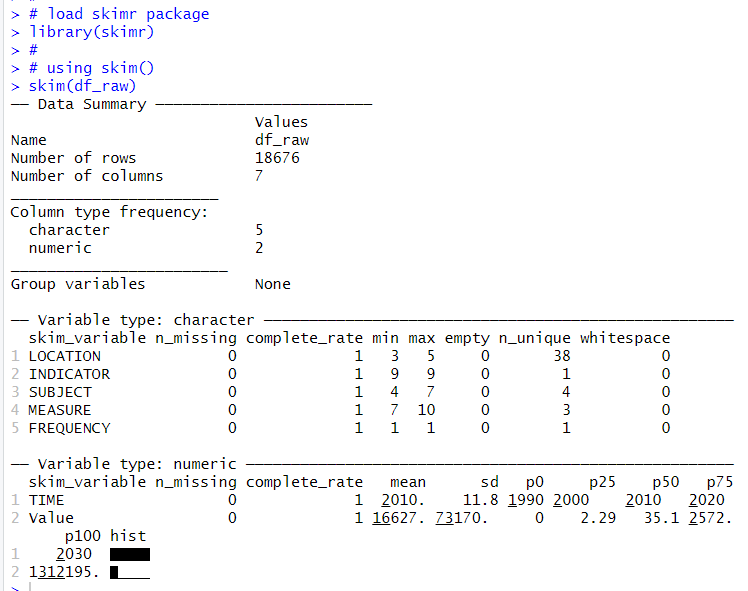I see there are 5 character variables and 2 numerical vatiables in "df_raw" object and there is not any missing observations.

Let's see LOCATION using table() function.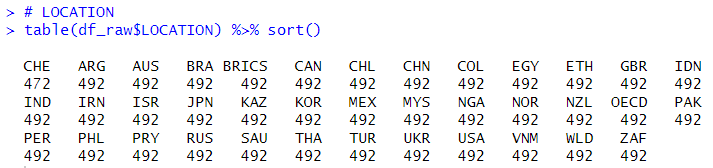Only CHE has 472 observations and others have 492 observations. So I delete CHE.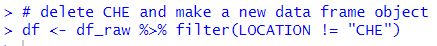Next, let's see INDICATOR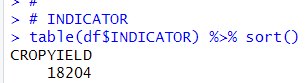INDICATOR has only one value, CROPYIELD. I remove INDICATOR.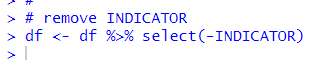Next, let's see SUBJECT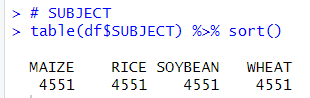There 4 values, MAIZE, RICE, SOYBEAN and WHEAT. They have the same number of observations, 4551.

Next, let's see MEASURE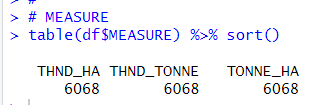Thare are three values,

THND_HA means thousand hectares,

THND_TONNE means thousand tonnes,

TONNE_HA means tonnes / hectare.

Next, let's see FREQENCY.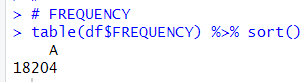FREQUEANCY has only one value, so I remove FREQUENCY.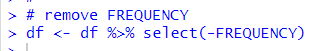Next, let' see TIME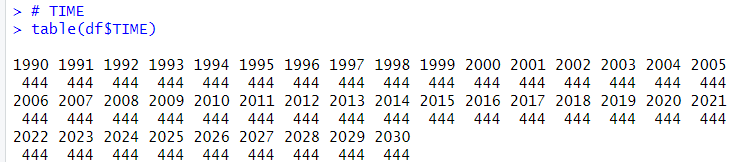TIME starts from 1900 and 2030, they are 444 observations each year.

Lastly, let's see Value. I want see basic stats for each SUNJECT x MEASURE.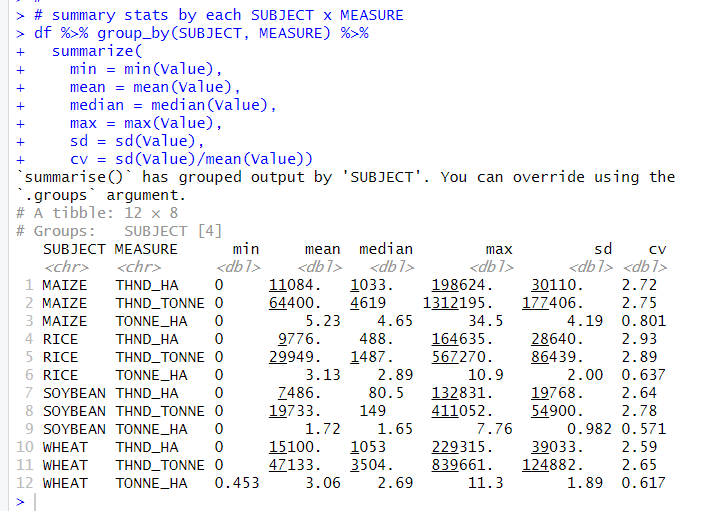For THND_HA, it is area of fields, WHEAT has the largest mean.
For THND_TONEE, it is volume of crops, MAIZE has the largest mean.
For TONNE_HA, it is volume / area, MAIZE has the largest mean.

That's it. Thank you!

Next post is